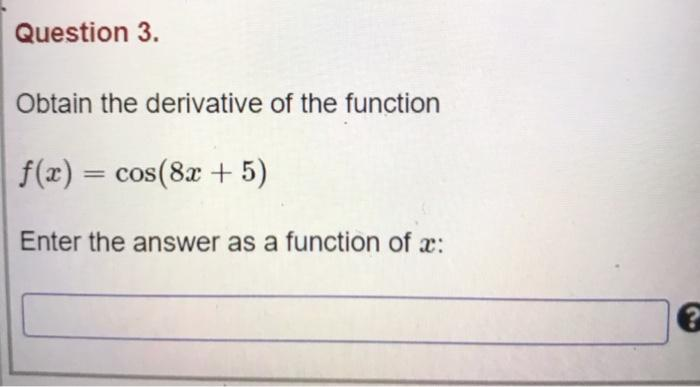### Create an Account

Already have account?

### Forgot Your Password ?

Home / Questions / Question 3. Obtain the derivative of the function f(x) = cos(8x + 5) Enter the answer as a...

# Question 3. Obtain the derivative of the function f(x) = cos(8x + 5) Enter the answer as a function of c:

Question 3. Obtain the derivative of the function f(x) = cos(8x + 5) Enter the answer as a function of c:Apr 14 2021 View more View Less

#### Answer (Solved)Subscribe To Get Solution